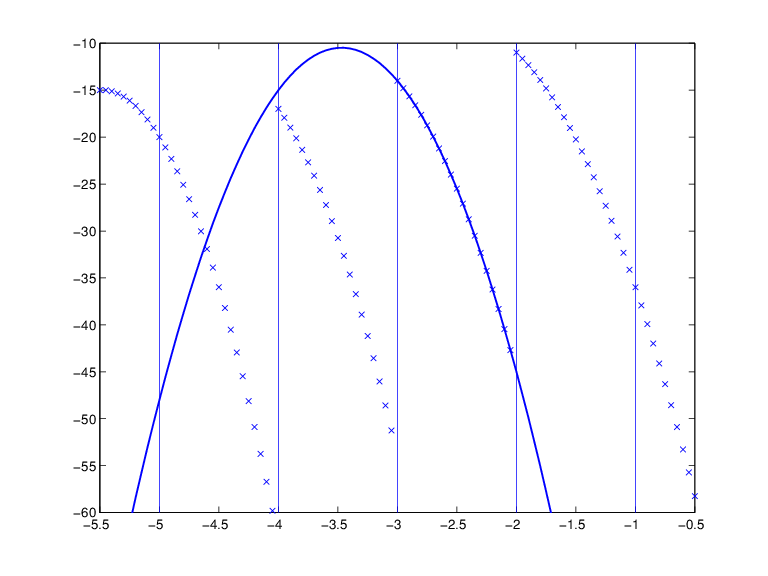## Constructing and Working with ppform Splines

### Constructing a ppform

A piecewise-polynomial is usually constructed by some command, through a process of interpolation or approximation, or conversion from some other form e.g., from the B-form, and is output as a variable. But it is also possible to make one up from scratch, using the statement

```pp = ppmak(breaks,coefs) ```

For example, if you enter `pp=ppmak(-5:-1,-22:-11)`, or, more explicitly,

```breaks = -5:-1; coefs = -22:-11; pp = ppmak(breaks,coefs); ```

you specify the uniform break sequence -`5:`-`1` and the coefficient sequence -`22:`-`11`. Because this break sequence has 5 entries, hence 4 break intervals, while the coefficient sequence has 12 entries, you have, in effect, specified a piecewise-polynomial of order 3 (= 12/4). The command

`fnbrk(pp) `

prints out all the constituent parts of this piecewise-polynomial, as follows:

```breaks(1:l+1) -5 -4 -3 -2 -1 coefficients(d*l,k) -22 -21 -20 -19 -18 -17 -16 -15 -14 -13 -12 -11 pieces number l 4 order k 3 dimension d of target 1 ```

Further, `fnbrk` can be used to supply each of these parts separately. But the point of Curve Fitting Toolbox™ spline functionality is that you usually need not concern yourself with these details. You simply use `pp` as an argument to commands that evaluate, differentiate, integrate, convert, or plot the piecewise-polynomial whose description is contained in `pp`.

### Working With ppform Splines

Here are some functions for operations you can perform on a piecewise-polynomial.

 `v = fnval(pp,x)` Evaluates `dpp = fnder(pp)` Differentiates `dirpp = fndir(pp,dir)` Differentiates in the direction `dir` `ipp = fnint(pp)` Integrates `fnmin(pp,[a,b])` Finds the minimum value in given interval `fnzeros(pp,[a,b])` Finds the zeros in the given interval `pj = fnbrk(pp,j)` Pulls out the `j`th polynomial piece `pc = fnbrk(pp,[a b])` Restricts/extends to the interval [`a`..`b`] `po = fnxtr(pp,order)` Extends outside its basic interval by polynomial of specified order `fnplt(pp,[a,b])` Plots on given interval `sp = fn2fm(pp,'B-') ` Converts to B-form `pr = fnrfn(pp,morebreaks) ` Inserts additional breaks

Inserting additional breaks comes in handy when you want to add two piecewise-polynomials with different breaks, as is done in the command `fncmb`.

### Example ppform

Execute the following commands to create and plot the particular piecewise-polynomial (ppform) described in the Constructing a ppform section.

1. Create the piecewise-polynomial with break sequence `-5:-1` and coefficient sequence `-22:-11`:

`pp=ppmak(-5:-1,-22:-11)`
2. Create the basic plot:

```x = linspace(-5.5,-.5,101); plot(x, fnval(pp,x),'x') ```
3. Add the break lines to the plot:

```breaks=fnbrk(pp,'b'); yy=axis; hold on for j=1:fnbrk(pp,'l')+1 plot(breaks([j j]),yy(3:4)) end ```
4. Superimpose the plot of the polynomial that supplies the third polynomial piece:

```plot(x,fnval(fnbrk(pp,3),x),'linew',1.3) set(gca,'ylim',[-60 -10]), hold off ```

A Piecewise-Polynomial Function, Its Breaks, and the Polynomial Giving Its Third PieceThe figure above is the final picture. It shows the piecewise-polynomial as a sequence of points and, solidly on top of it, the polynomial from which its third polynomial piece is taken. It is quite noticeable that the value of a piecewise-polynomial at a break is its limit from the right, and that the value of the piecewise-polynomial outside its basic interval is obtained by extending its leftmost, respectively its rightmost, polynomial piece.

While the ppform of a piecewise-polynomial is efficient for evaluation, the construction of a piecewise-polynomial from some data is usually more efficiently handled by determining first its B-form, i.e., its representation as a linear combination of B-splines.